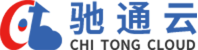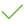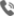UI设计定制 个性化定制及视觉设计、为企业节省开发成本 提供高质量的维护服务PC站/手机站/微官网一个都不能少全部个性化视觉设计 统一品牌形象适应 多种终端方便用户浏览

/
4800
2600
5200
15800
21800
23888
33888

10+
20+
30+
40+
50+
60+
70+
80+

2600
5200
7800
10500
2600
5200
18000
20800

90+
160+
360+
480+
210+
280+
520+
680+

100+
200+
300+
400+
500+
600+
700+
800+

50+
100+
150+
200+
210+
280+
350+
400+

10+
20+
30+
40+
50+
60+
70+
80+

3
4
5
6
7
8
9
10
Banner图

2
3
3
4
4
5
5
6

6
7
7
8
8
9
9
10

/
/1号视频

/
/
/
/V.line：86-851-88565888